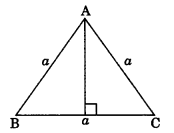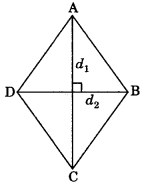On this page, you will find Heron’s Formula Class 9 Notes Maths Chapter 7 Pdf free download. CBSE NCERT Class 9 Maths Notes Chapter 7 Heron’s Formula will seemingly help them to revise the important concepts in less time.

## CBSE Class 9 Maths Chapter 7 Notes Heron’s Formula

### Heron’s Formula Class 9 Notes Understanding the Lesson

1. Area of triangle with base ‘b’ and altitude ‘h’ is
Area = $$\frac{1}{2}$$(b x h)2. Area of an isosceles triangle with equal sides ‘a’ each and third side b is
Area $$=\frac{b}{4} \sqrt{4 a^{2}-b^{2}}$$3. Area of an equilateral triangle with side ‘a’ each is
Area=$$\frac{\sqrt{3}}{4} a^{2}$$4. Area of a triangle by Heron’s formula when sides a, b and c are given is
Area = $$\sqrt{s(s-a)(s-b)(s-c)}$$
Where s = semi-perimeter = \frac{a+b+c}{2}5. Area of rhombus
Area= $$\frac{1}{2} d_{1} \times d_{2}$$
where d1 and d2 are the lengths of its diagonals.6. Area of trapezium
Area=$$\frac{1}{2}$$ (a+b) h
where a and b are parallel sides and h is distance between two parallel sides.